Next: Defining an Affine Restriction Up: From Projective to Affine Previous: From Projective to Affine

## The Need for Affine Space

Projective geometry allows us to discuss coplanarity, and relative position using the cross ratio or its derivatives. However in standard projective space there is no consistent notion of betweenness. For instance, we can not uniquely define the line segment linking two points A, B. The problem is that projective lines are topologically circular: they close on themselves when they pass through infinity (except that infinity is not actually distinguished in projective space -- all points of the line are equal). So there are two equally valid segments linking two points on a projective line: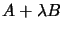for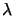in either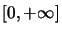and in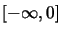.

One solution to this problem is to distinguish a set (in fact a hyperplane) of points at infinity in projective space: this gives us affine space.

A related problem occurs if we try to define the convex hull of a set of points. Figure 4.1 illustrates the behavior of the convex hull for two different affine interpretations of the plane, i.e. for two different choices of the line at infinity. In the first case, the line at infinity (the ideal line) does not pass through the intuitive convex hull (fig. 4.1a), so the convex hull remains similar to the intuitive one. But in figure 4.1b the line cuts the intuitive convex hull, so Band C turn out to be inside the calculated hull in this case. (No side of the calculated convex hull ever cuts the line at infinity). Note that in this case, we need only know whether the ideal line passes through the desired convex hull or not; this naturally extends to the ideal plane in the 3D space (see ).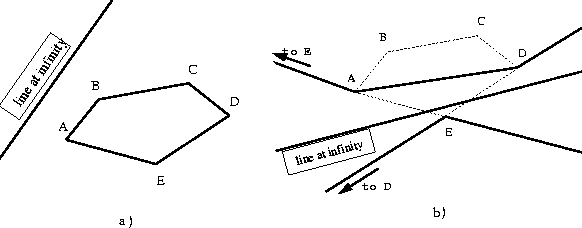Another important limitation when working with projective geometry is that it does not allow us to define the midpoint of a segment: the midpoint is defined by a simple ratio and projective geometry deals only with cross ratios.Next: Defining an Affine Restriction Up: From Projective to Affine Previous: From Projective to Affine
Bill Triggs
1998-11-13